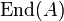# Endomorphism ring of an abelian group

(diff) ← Older revision | Latest revision (diff) | Newer revision → (diff)
Suppose$A$ is an abelian group. The endomorphism ring of$A$, denoted$\operatorname{End}(A)$ is defined as follows:
1. As a set, it is the set of all endomorphisms of$A$.
2. The addition is pointwise addition in the target group. In other words, for endomorphisms$f,g$ of$A$, we define$f + g$ as the map$a \mapsto f(a) + g(a)$. Thus, the additive identity is the zero map (the map sending everything to zero) and the negation is the pointwise negation.
3. The multiplication is given by function composition. In other words,$fg$ is the map sending$a$ to$f(g(a))$. The identity for multiplication is the identity map.